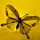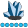## What happen with Do j=i-1 to j=i-3.

Hi,

I know the correct syntax should be

``do j=i-1 to i-3 by -1 until (found=1);``

`do j=i-1 to j=i-3 by -1 until (found=1);`

However, SAS still run this command and I don't know what really happen there.

Look like SAS will run all the way to the end of data.

I wonder why SAS doesn't give at least a warning?

Thanks,

HHC

``````data have;
input id t value;
datalines;
1 1 2
1 2 5
1 3 6
1 4 7
1 5 8
1 6 9
;run;

data want;
set have;
i+1;
if value=8 then do;
found=0;
count=0;
do j=i-1 to j=i-3 by -1 until (found=1);
set have (keep=t value rename=(t = tt value=v)) point=j;
count=count+1;
if v=1 then do;
target=tt;
found=1;
end;
end;
end;
run;``````

1 ACCEPTED SOLUTION

Accepted Solutions

## Re: What happen with Do j=i-1 to j=i-3.

Consider how SAS evaluates this statement:

``do j=i-1 to j=i-3 by -1 until (found=1);``

Here, "j=i-3" is a logical expression, which SAS automatically evaluates.  Evaluating a logical expression yields 0 if the expression is false, or 1 if the expression is true.  So the software evaluates this as one of these two possibilities:

``````do j=i-1 to 0 by -1 until (found=1);

do j=i-1 to 1 by -1 until (found=1);``````

More specifically, j begins with a missing value.  But i will not be missing since incrementing with the statement i+1; assigns a nonmissing value to i.  So the software executes this as:

``do j=i-1 to 0 by -1 until (found=1);``

Some SAS puzzles have been built around this theme of logical expressions, using a statement such as:

``do j=1 to 5, i=4 to 6;``

How many times should that loop execute, and with what values for j and i ?

11 REPLIES 11

## Re: What happen with Do j=i-1 to j=i-3.

Consider how SAS evaluates this statement:

``do j=i-1 to j=i-3 by -1 until (found=1);``

Here, "j=i-3" is a logical expression, which SAS automatically evaluates.  Evaluating a logical expression yields 0 if the expression is false, or 1 if the expression is true.  So the software evaluates this as one of these two possibilities:

``````do j=i-1 to 0 by -1 until (found=1);

do j=i-1 to 1 by -1 until (found=1);``````

More specifically, j begins with a missing value.  But i will not be missing since incrementing with the statement i+1; assigns a nonmissing value to i.  So the software executes this as:

``do j=i-1 to 0 by -1 until (found=1);``

Some SAS puzzles have been built around this theme of logical expressions, using a statement such as:

``do j=1 to 5, i=4 to 6;``

How many times should that loop execute, and with what values for j and i ?whymath
Lapis Lazuli | Level 10

## Re: What happen with Do j=i-1 to j=i-3.

Amazing trick! Does sombody really use it in reality?

## Re: What happen with Do j=i-1 to j=i-3.

This particular trick?  No.  But even beginning programmers use logical expressions all the time.  For example, consider:

``if first.state then do;``

There is no comparison there.  First.state is either 1 or 0, and the software considers 1 to be true and 0 to be false.  So there is no need for:

``if first.state=1 then do;``

Actually, the evaluation of logical expressions goes beyond 1 and 0.  The software considers 0 and missing values to be false, and all other values (including negative numbers) to be true.  So consider a simple statement:

``a = b / c;``

When c is 0, the software notes that division by zero took place, and tracks how many times it happened.   It also runs up the bill, taking vastly more CPU time.  Similarly, when c is missing, the result is that a missing value gets generated.  Again, the software runs up the bill (CPU time, that is), with no usable numeric result.  So if you have lots of missing values of zeros for C, it can be faster to use this statement:

``if c then a = b / c;``

The IF clause treats C as a logical expression (false for missings and zero, but true for all other values.  So there is no attempt to calculate anything when C is missing or zero.  I don't think I can come up with a realistic use for:

``do i=1 to 5, j=5 to 7;``whymath
Lapis Lazuli | Level 10

## Re: What happen with Do j=i-1 to j=i-3.

You really study SAS in deeply, thank you, Astounding.

## Re: What happen with Do j=i-1 to j=i-3.

@whymath wrote:
Amazing trick! Does sombody really use it in reality?

Intentionally? I would say only by those who specialize in writing obscure code in the hopes of job security as no one else could follow it easily. 😈whymath
Lapis Lazuli | Level 10

## Re: What happen with Do j=i-1 to j=i-3.

Agree with you. I may use it in code golf game but not in production.

## Re: What happen with Do j=i-1 to j=i-3.

@Astounding wrote:

Some SAS puzzles have been built around this theme of logical expressions, using a statement such as:

``do j=1 to 5, i=4 to 6;``

How many times should that loop execute, and with what values for j and i ?

The Puzzle Master casually drops a masterpiece.  @yabwon, maybe steal this for a #sasensei question.

Check out the Boston Area SAS Users Group (BASUG) video archives: https://www.basug.org/videos.

## Re: What happen with Do j=i-1 to j=i-3.

@Quentin  That question would be a sneaky one! 🙂

_______________
Polish SAS Users Group: www.polsug.com and communities.sas.com/polsug

"SAS Packages: the way to share" at SGF2020 Proceedings (the latest version), GitHub Repository, and YouTube Video.
Hands-on-Workshop: "Share your code with SAS Packages"
"My First SAS Package: A How-To" at SGF2021 Proceedings

SAS Ballot Ideas: one: SPF in SAS, two, and three
SAS Documentation

## Re: What happen with Do j=i-1 to j=i-3.

For the 5th observation, you have i=5 and value=8, which activates this loop:

``    do j=i-1 to j=i-3 by -1 until (found=1);``

J starts the loop at 4, as expected, but does not end at J=2.  Instead, it ends at J=0 (more on that below), when the statement

``set have (keep=t value rename=(t = tt value=v)) point=j;``

attempts to read the non-existent observation 0, at which point the automatic variable _ERROR_ is set to 1 for that observation.

Now, why doesn't the loop

``    do j=i-1 to j=i-3 by -1 until (found=1);``

stop at J=2 when i=5?

I see no ready answer to that question.  The SET statement with the "point=j" option reads obs 4, then obs 3, all the way to the failed obs 0 (I tested this by putting a "put j=;" statement after the SET statement).

This sequence happens even if I change the end condition from j=i-3 to, say j=i-1, as in:

``    do j=i-1 to j=i-1 by -1 until (found=1);``

It doesn't stop any earlier.  It keep SETting obs until the _ERROR_=1 condition is generated by pointing at obs zero.

But lest you think generating _ERROR_=1 is always enough to stop a loop containing a SET .... POINT= statement, then type

``    do j=i-1 to (j=i)-3 by -1 until (found=1);``

This will make attempts to read obs -1, -2, -3, even after _ERROR_=1 due to failed read of obs 0.  That's "logical" since (j=i) is always 0, so (j-1)-3 is minus 3.

So the _ERROR_=1 condition seems to selectively stop the loop iteration.

--------------------------
The hash OUTPUT method will overwrite a SAS data set, but not append. That can be costly. Consider voting for Add a HASH object method which would append a hash object to an existing SAS data set

Would enabling PROC SORT to simultaneously output multiple datasets be useful? Then vote for
Allow PROC SORT to output multiple datasets

--------------------------

## Re: What happen with Do j=i-1 to j=i-3.

Try it and see for yourself.

``````data _null_;
do i=0 to 5;
put i= @;
do j=i-1 to j=i-3 by -1 ;
put j= @ ;
end;
put;
end;
run;
``````
Spoiler
```28   data _null_;
29     do i=0 to 5;
30       put i= @;
31       do j=i-1 to j=i-3 by -1 ;
32         put j= @ ;
33       end;
34       put;
35     end;
36   run;

i=0
i=1 j=0
i=2 j=1
i=3 j=2 j=1
i=4 j=3 j=2 j=1 j=0
i=5 j=4 j=3 j=2 j=1 j=0
```

The first thing to notice is this is the exact same result you get if you add in some grouping to control the order of operations.

``do j=i-1 to j=(i-3) by -1 ;``

So let's show all of the values being evaluated here and see how the change as J is decremented.  We have the value I,  And the value of (I-3).  Those do not change as the loop progresses.  Then J starts at I-1 and decrements by one after each "run".  To decide whether to run the do loop it needs to compare if the value of J is less than the value of J=(i-3).

Spoiler

```I  (i-3)   J  j=(i-3) J too small?
0   -3    -1    0     STOP

1   -2     0    0     RUN
1   -2    -1    0     STOP

2    0     1    0     RUN
2    0     0    1     STOP

3    0     2    0     RUN
3    0     1    0     RUN
3    0     0    1     STOP

4    1     3    0     RUN
4    1     2    0     RUN
4    1     1    1     RUN
4    1     0    0     RUN
4    1    -1    0     STOP
```

## Re: What happen with Do j=i-1 to j=i-3.

The DO-Loop in it's most general form (according to the documentation) is:

``````DO index-variable
=  start_expression1 <TO stop_expression1><BY increment_expression1> <WHILE(expression1)| UNTIL(expression1)>
<, start_expression2 <TO stop_expression2><BY increment_expression2> <WHILE(expression2)| UNTIL(expression2)>>
...
<, start_expressionN <TO stop_expressionN><BY increment_expressionN> <WHILE(expressionN)| UNTIL(expressionN)>>
;

...moreSAS statements...

END;``````

<> - means optional element

| - means exclusive or

``do j=i-1 to j=i-3 by -1 until (found=1);``

is perfectly good example of a do-loop with

start expression:

``i-1``

stop expression:

``j=i-3``

by expression:

``-1``

and until condition:

``found=1``

And, as others wrote, you will get the loop from i-1 to 0 or 1 (depends how the stop expression evaluates) by -1.

By the  way  looping with  point wont give you any warning even for negative values, you will get only one  "put log" for _error_=1 at the very end of processing:

``````1    data x;
2      do point=-1,-2,-3,-4,-5,-6,-7;
3        set sashelp.class point=point;
4        output;
5      end;
6
7      stop;
8    run;

point=-7 Name=  Sex=  Age=. Height=. Weight=. _ERROR_=1 _N_=1
NOTE: The data set WORK.X has 7 observations and 5 variables.
NOTE: DATA statement used (Total process time):
real time           0.01 seconds
cpu time            0.01 seconds
``````

Doc. only states:

 If SAS reads an invalid value of the POINT= variable, it sets the automatic variable _ERROR_ to 1.

Thinking on where such "conditional" loop could be used, for short list of variables cumulative condition like below could be a use case:

``````resetline;
data have;
input a b c;
cards;
1 2 3
4 5 6
7 8 9
;
run;

data want;
set have;
do i=a>5, b>5, c>5;
cnt+i;
end;
run;
proc print;
run;``````

Bart

_______________
Polish SAS Users Group: www.polsug.com and communities.sas.com/polsug

"SAS Packages: the way to share" at SGF2020 Proceedings (the latest version), GitHub Repository, and YouTube Video.
Hands-on-Workshop: "Share your code with SAS Packages"
"My First SAS Package: A How-To" at SGF2021 Proceedings

SAS Ballot Ideas: one: SPF in SAS, two, and three
SAS Documentation

Discussion stats
• 11 replies
• 482 views
• 9 likes
• 8 in conversation